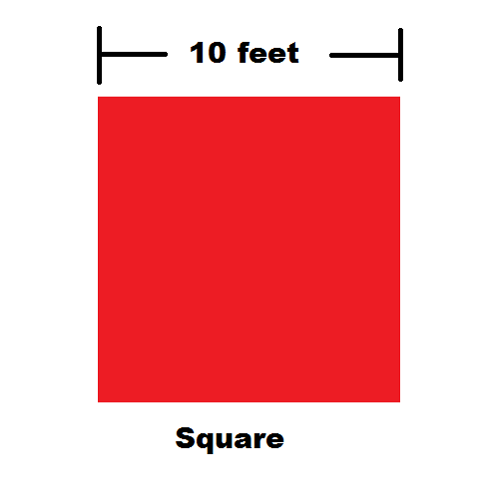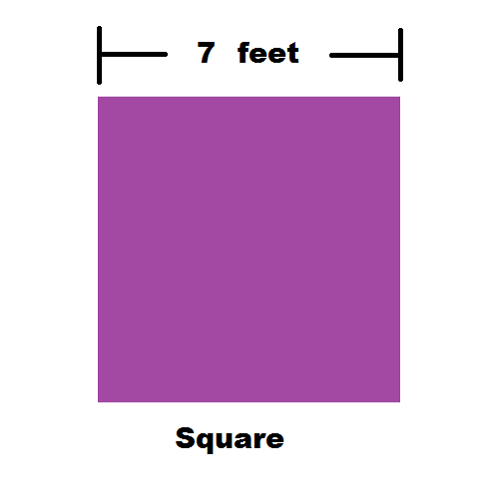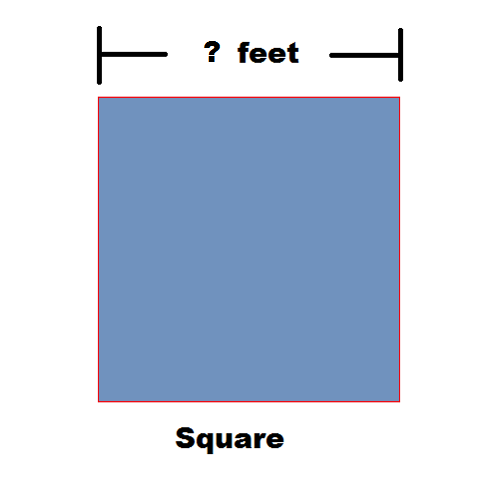# Perimeter & Area of a Square

Instructions:

question 1 of 3

### What is the perimeter of this square?Create Your Account To Take This Quiz

As a member, you'll also get unlimited access to over 79,000 lessons in math, English, science, history, and more. Plus, get practice tests, quizzes, and personalized coaching to help you succeed.

Try it risk-free for 30 days. Cancel anytime

### 1. What is the area of this square?### 2. If the side of this square is 3 feet, how long is the perimeter?Create your account to access this entire worksheet
Quizzes, practice exams & worksheets
Certificate of Completion
Create an account to get started

This quiz and worksheet test and review your understanding of area and perimeter of a square. You'll review how to find the area and the perimeter and test your ability with some sample problems to solve.

## Quiz & Worksheet Goals

The purpose of this quiz and worksheet is to offer a review of:

• How to find the area of a square
• What the perimeter is
• Finding the area or perimeter with only one side of a square

## Skills Practiced

• Information recall - see if you can remember basic rules of squares that allow you to figure out the perimeter or area with only one side's measurement
• Knowledge application - review what you know about the perimeter and area to answer questions about how to find them
• Problem solving - use what you know about squares to solve for the area or perimeter

Review this topic in greater detail with the provided lesson, Perimeter & Area of a Square. This lesson offers more details about:

• Using a real-world example
• The definition and formula for the area of a square
• What perimeter is and how to calculate it
Final ExamMath for Kids
Status: Not Started
Chapter ExamShapes for Elementary School
Status: Not Started

Support